# Performance of Sparse Recovery Using L1 Minimization¶

Important: Please read the installation page for details about how to install the toolboxes. $\newcommand{\dotp}{\langle #1, #2 \rangle}$ $\newcommand{\enscond}{\lbrace #1, #2 \rbrace}$ $\newcommand{\pd}{ \frac{ \partial #1}{\partial #2} }$ $\newcommand{\umin}{\underset{#1}{\min}\;}$ $\newcommand{\umax}{\underset{#1}{\max}\;}$ $\newcommand{\umin}{\underset{#1}{\min}\;}$ $\newcommand{\uargmin}{\underset{#1}{argmin}\;}$ $\newcommand{\norm}{\|#1\|}$ $\newcommand{\abs}{\left|#1\right|}$ $\newcommand{\choice}{ \left\{ \begin{array}{l} #1 \end{array} \right. }$ $\newcommand{\pa}{\left(#1\right)}$ $\newcommand{\diag}{{diag}\left( #1 \right)}$ $\newcommand{\qandq}{\quad\text{and}\quad}$ $\newcommand{\qwhereq}{\quad\text{where}\quad}$ $\newcommand{\qifq}{ \quad \text{if} \quad }$ $\newcommand{\qarrq}{ \quad \Longrightarrow \quad }$ $\newcommand{\ZZ}{\mathbb{Z}}$ $\newcommand{\CC}{\mathbb{C}}$ $\newcommand{\RR}{\mathbb{R}}$ $\newcommand{\EE}{\mathbb{E}}$ $\newcommand{\Zz}{\mathcal{Z}}$ $\newcommand{\Ww}{\mathcal{W}}$ $\newcommand{\Vv}{\mathcal{V}}$ $\newcommand{\Nn}{\mathcal{N}}$ $\newcommand{\NN}{\mathcal{N}}$ $\newcommand{\Hh}{\mathcal{H}}$ $\newcommand{\Bb}{\mathcal{B}}$ $\newcommand{\Ee}{\mathcal{E}}$ $\newcommand{\Cc}{\mathcal{C}}$ $\newcommand{\Gg}{\mathcal{G}}$ $\newcommand{\Ss}{\mathcal{S}}$ $\newcommand{\Pp}{\mathcal{P}}$ $\newcommand{\Ff}{\mathcal{F}}$ $\newcommand{\Xx}{\mathcal{X}}$ $\newcommand{\Mm}{\mathcal{M}}$ $\newcommand{\Ii}{\mathcal{I}}$ $\newcommand{\Dd}{\mathcal{D}}$ $\newcommand{\Ll}{\mathcal{L}}$ $\newcommand{\Tt}{\mathcal{T}}$ $\newcommand{\si}{\sigma}$ $\newcommand{\al}{\alpha}$ $\newcommand{\la}{\lambda}$ $\newcommand{\ga}{\gamma}$ $\newcommand{\Ga}{\Gamma}$ $\newcommand{\La}{\Lambda}$ $\newcommand{\si}{\sigma}$ $\newcommand{\Si}{\Sigma}$ $\newcommand{\be}{\beta}$ $\newcommand{\de}{\delta}$ $\newcommand{\De}{\Delta}$ $\newcommand{\phi}{\varphi}$ $\newcommand{\th}{\theta}$ $\newcommand{\om}{\omega}$ $\newcommand{\Om}{\Omega}$

This tour explores theoritical garantees for the performance of recovery using $\ell^1$ minimization.

In :
using PyPlot
using NtToolBox
# arequire("NtToolBox")


## Sparse $\ell^1$ Recovery¶

We consider the inverse problem of estimating an unknown signal $x_0 \in \RR^N$ from noisy measurements $y=\Phi x_0 + w \in \RR^P$ where $\Phi \in \RR^{P \times N}$ is a measurement matrix with $P \leq N$, and $w$ is some noise.

This tour is focused on recovery using $\ell^1$ minimization $$x^\star \in \uargmin{x \in \RR^N} \frac{1}{2}\norm{y-\Phi x}^2 + \la \norm{x}_1.$$

Where there is no noise, we consider the problem $\Pp(y)$ $$x^\star \in \uargmin{\Phi x = y} \norm{x}_1.$$

We are not concerned here about the actual way to solve this convex problem (see the other numerical tours on sparse regularization) but rather on the theoritical analysis of wether $x^\star$ is close to $x_0$.

More precisely, we consider the following three key properties

• Noiseless identifiability: $x_0$ is the unique solution of $\Pp(y)$ for $y=\Phi x_0$.
• Robustess to small noise: one has $\norm{x^\star - x_0} = O(\norm{w})$ for $y=\Phi x_0+w$ if $\norm{w}$ is smaller than an arbitrary small constant that depends on $x_0$ if $\la$ is well chosen according to $\norm{w}$.
• Robustess to bounded noise: same as above, but $\norm{w}$ can be arbitrary.

Note that noise robustness implies identifiability, but the converse is not true in general.

## Coherence Criteria¶

The simplest criteria for identifiality are based on the coherence of the matrix $\Phi$ and depends only on the sparsity $\norm{x_0}_0$ of the original signal. This criteria is thus not very precise and gives very pessimistic bounds.

The coherence of the matrix $\Phi = ( \phi_i )_{i=1}^N \in \RR^{P \times N}$ with unit norm colum $\norm{\phi_i}=1$ is $$\mu(\Phi) = \umax{i \neq j} \abs{\dotp{\phi_i}{\phi_j}}.$$

Compute the correlation matrix (remove the diagonal of 1's).

In :
remove_diag = C -> C - diagm(diag(C))
Correlation = Phi -> remove_diag(abs(Phi'*Phi));


Compute the coherence $\mu(\Phi)$.

In :
mu = Phi -> maximum(Correlation(Phi));


The condition $$\norm{x_0}_0 < \frac{1}{2}\pa{1 + \frac{1}{\mu(\Phi)}}$$ implies that $x_0$ is identifiable, and also implies to robustess to small and bounded noise.

Equivalently, this condition can be written as $\text{Coh}(\norm{x_0}_0)<1$ where $$\text{Coh}(k) = \frac{k \mu(\Phi)}{ 1 - (k-1)\mu(\Phi) }$$

In :
Coh = (Phi, k) -> (k*mu(Phi))/(1 - (k - 1)*mu(Phi));


Generate a matrix with random unit columns in $\RR^P$.

In :
normalize = Phi -> Phi ./ repeat(sqrt(sum(Phi.^2, 1)), inner = [size(Phi), 1]);

PhiRand = (P, N) -> normalize(randn(P, N))
Phi = PhiRand(250, 1000);


Compute the coherence and the maximum possible sparsity to ensure recovery using the coherence bound.

In :
c = mu(Phi)
println(@sprintf("Coherence:    %.2f", c))
println(@sprintf("Sparsity max: %d", floor(1/2*(1 + 1/c))))

Coherence:    0.31
Sparsity max: 2


Exercise 1

Display how the average coherence of a random matrix decays with the redundancy $\eta = P/N$ of the matrix $\Phi$. Can you derive an empirical law between $P$ and the maximal sparsity?

In :
include("NtSolutions/sparsity_6_l1_recovery/exo1.jl")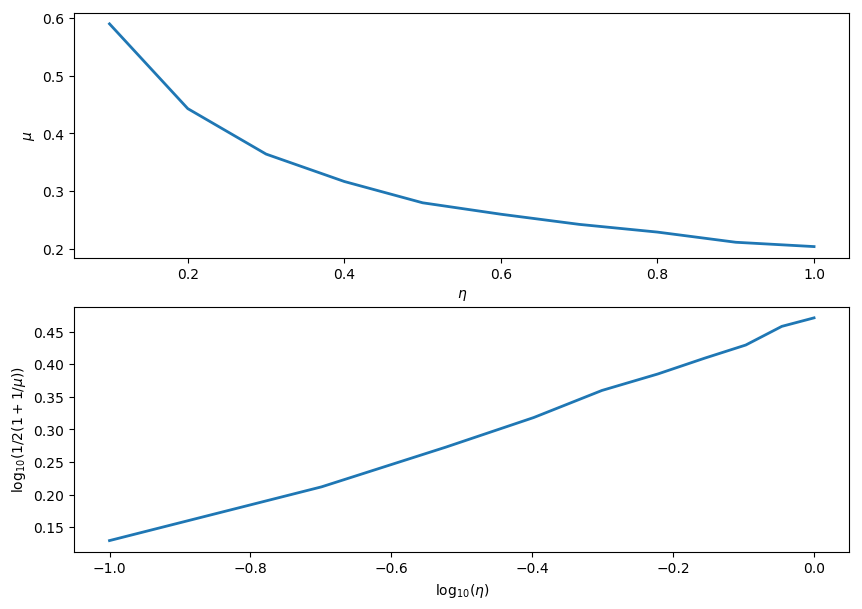In :
## Insert your code here.


## Support and Sign-based Criteria¶

In the following we will consider the support $$\text{supp}(x_0) = \enscond{i}{x_0(i) \neq 0}$$ of the vector $x_0$. The co-support is its complementary $I^c$.

In :
supp   = s -> find(abs(s) .> 1e-5)
cosupp = s -> find(abs(s) .< 1e-5);


Given some support $I \subset \{0,\ldots,N-1\}$, we will denote as $\Phi = (\phi_m)_{m \in I} \in \RR^{N \times \abs{I}}$ the sub-matrix extracted from $\Phi$ using the columns indexed by $I$.

J.J. Fuchs introduces a criteria $F$ for identifiability that depends on the sign of $x_0$.

J.J. Fuchs. Recovery of exact sparse representations in the presence of bounded noise. IEEE Trans. Inform. Theory, 51(10), p. 3601-3608, 2005

Under the condition that $\Phi_I$ has full rank, the $F$ measure of a sign vector $s \in \{+1,0,-1\}^N$ with $\text{supp}(s)=I$ reads $$\text{F}(s) = \norm{ \Psi_I s_I }_\infty \qwhereq \Psi_I = \Phi_{I^c}^* \Phi_I^{+,*}$$ where $A^+ = (A^* A)^{-1} A^*$ is the pseudo inverse of a matrix $A$.

The condition $$\text{F}(\text{sign}(x_0))<1$$ implies that $x_0$ is identifiable, and also implies to robustess to small noise. It does not however imply robustess to a bounded noise.

Compute $\Psi_I$ matrix.

In :
PsiI = (Phi,I) -> Phi[:, setdiff(1:size(Phi), I)]' * pinv(Phi[:,I])';


Compute $\text{F}(s)$.

In :
F = (Phi, s) -> norm(PsiI(Phi, supp(s))*s[supp(s)], Inf);


The Exact Recovery Criterion (ERC) of a support $I$, introduced by Tropp in

J. A. Tropp. Just relax: Convex programming methods for identifying sparse signals. IEEE Trans. Inform. Theory, vol. 52, num. 3, pp. 1030-1051, Mar. 2006.

Under the condition that $\Phi_I$ has full rank, this condition reads $$\text{ERC}(I) = \norm{\Psi_{I}}_{\infty,\infty} = \umax{j \in I^c} \norm{ \Phi_I^+ \phi_j }_1.$$ where $\norm{A}_{\infty,\infty}$ is the $\ell^\infty-\ell^\infty$ operator norm of a matrix $A$.

In :
erc =(Phi, I) -> norm(PsiI(Phi,I), Inf);


The condition $$\text{ERC}(\text{supp}(x_0))<1$$ implies that $x_0$ is identifiable, and also implies to robustess to small and bounded noise.

One can prove that the ERC is the maximum of the F criterion for all signs of the given support $$\text{ERC}(I) = \umax{ s, \text{supp}(s) \subset I } \text{F}(s).$$

The weak-ERC is an approximation of the ERC using only the correlation matrix $$\text{w-ERC}(I) = \frac{ \umax{j \in I^c} \sum_{i \in I} \abs{\dotp{\phi_i}{\phi_j}} }{ 1-\umax{j \in I} \sum_{i \neq j \in I} \abs{\dotp{\phi_i}{\phi_j}} }$$

In :
g = (C, I) -> sum(C[:,I], 2)
werc_g = (g, I, J) -> maximum(g[J]) / (1 - maximum(g[I]))
werc = (Phi, I) -> werc_g(g(Correlation(Phi), I), I, setdiff(1:size(Phi), I) );


One has, if $\text{w-ERC}(I)>0$, for $I = \text{supp}(s)$, $$\text{F}(s) \leq \text{ERC}(I) \leq \text{w-ERC}(I) \leq \text{Coh}(\abs{I}).$$

This shows in particular that the condition $$\text{w-ERC}(\text{supp}(x_0))<1$$ implies identifiability and robustess to small and bounded noise.

Exercise 2

Show that this inequality holds on a given matrix. What can you conclude about the sharpness of these criteria ?

In :
include("NtSolutions/sparsity_6_l1_recovery/exo2.jl")

N = 2000, P = 1990, |I| = 6
F(s)     = 0.18
ERC(I)   = 0.25
w-ERC(s) = 0.29
Coh(|s|) = 2.16

In :
## Insert your code here.

N = 2000
P = N - 10
Phi = PhiRand(N, P)
s = zeros(N)
s[1:6] = 1
I = supp(s)
k = length(I)

println(@sprintf("N = %d, P = %d, |I| = %d", N, P, k))
println(@sprintf("F(s)     = %.2f",  F(Phi, s)))
println(@sprintf("ERC(I)   = %.2f",  erc(Phi, I)))
println(@sprintf("w-ERC(s) = %.2f", werc(Phi, I)))
println(@sprintf("Coh(|s|) = %.2f", Coh(Phi, k)))

N = 2000, P = 1990, |I| = 6
F(s)     = 0.18
ERC(I)   = 0.23
w-ERC(s) = 0.25
Coh(|s|) = 1.65


Exercise 3

For a given matrix $\Phi$ generated using PhiRand, draw as a function of the sparsity $k$ the probability that a random sign vector $s$ of sparsity $\norm{s}_0=k$ satisfies the conditions $\text{F}(x_0)<1$, $\text{ERC}(x_0)<1$ and $\text{w-ERC}(x_0)<1$

In :
include("NtSolutions/sparsity_6_l1_recovery/exo3.jl")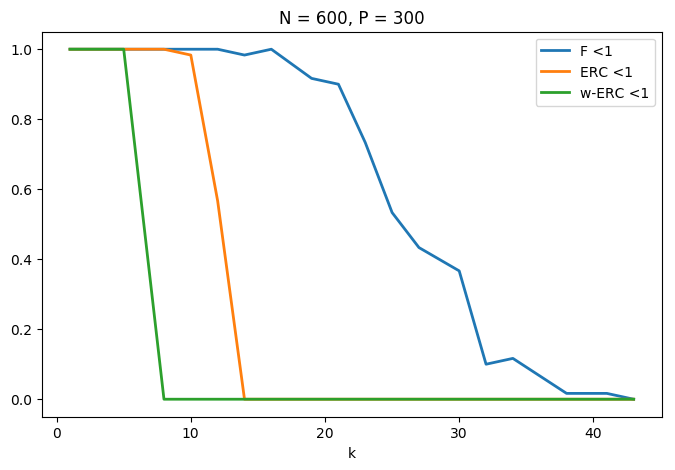In :
## Insert your code here.

N = 600
P = Int(N/2)
Phi = PhiRand(P, N)
klist = Array{Int64,1}(round(linspace(1, P/7., 20)))
ntrials = 60
proba = zeros(length(klist), 3)

for i in 1:length(klist)
proba[i, 1:3] = 0
k = Int(klist[i])
for j in 1:ntrials
s = zeros(N)
I = randperm(N)
I = I[1:k]
l = randn(k, 1)
s[I] = l./abs(l)
proba[i, 1] = proba[i, 1] + (F(Phi, s) .< 1)
proba[i, 2] = proba[i, 2] + (erc(Phi, I) .< 1)
proba[i, 3] = proba[i, 3] + (werc(Phi, I) .> 0).*(werc(Phi, I) .< 1)
end
end

figure(figsize = (8, 5))
plot(klist, proba/ntrials, linewidth = 2)
xlabel("k")
legend(["F <1", "ERC <1", "w-ERC <1"])
title(@sprintf("N = %d, P = %d", N, P))
show()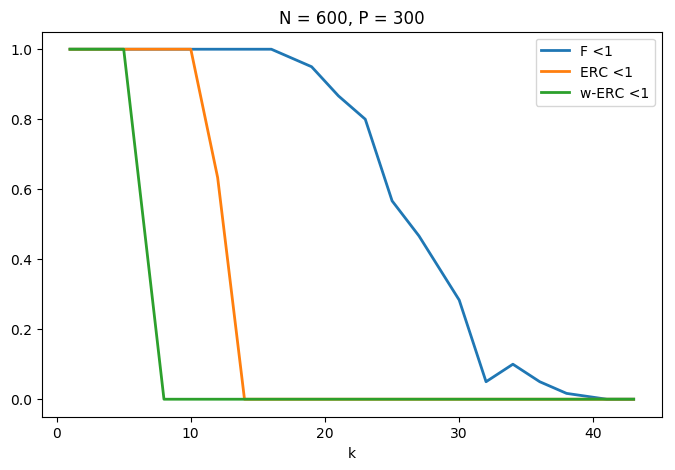## Restricted Isometry Criteria¶

The restricted isometry constants $\de_k^1,\de_k^2$ of a matrix $\Phi$ are the smallest $\de^1,\de^2$ that satisfy $$\forall x \in \RR^N, \quad \norm{x}_0 \leq k \qarrq (1-\de^1)\norm{x}^2 \leq \norm{\Phi x}^2 \leq (1+\de^2)\norm{x}^2.$$

E. Candes shows in

E. J. Cand s. The restricted isometry property and its implications for compressed sensing. Compte Rendus de l'Academie des Sciences, Paris, Serie I, 346 589-592

that if $$\de_{2k} \leq \sqrt{2}-1 ,$$ then $\norm{x_0} \leq k$ implies identifiability as well as robustness to small and bounded noise.

The stability constant $\la^1(A), \la^2(A)$ of a matrix $A = \Phi_I$ extracted from $\Phi$ is the smallest $\tilde \la_1,\tilde \la_2$ such that $$\forall \al \in \RR^{\abs{I}}, \quad (1-\tilde\la_1)\norm{\al}^2 \leq \norm{A \al}^2 \leq (1+\tilde \la_2)\norm{\al}^2.$$

These constants $\la^1(A), \la^2(A)$ are easily computed from the largest and smallest eigenvalues of $A^* A \in \RR^{\abs{I} \times \abs{I}}$

In :
minmax = v -> (1 - minimum(v), maximum(v) - 1)
ric = A -> minmax(eig(A'*A));


The restricted isometry constant of $\Phi$ are computed as the largest stability constants of extracted matrices $$\de^\ell_k = \umax{ \abs{I}=k } \la^\ell( \Phi_I ).$$

The eigenvalues of $\Phi$ are essentially contained in the interval $[a,b]$ where $a=(1-\sqrt{\be})^2$ and $b=(1+\sqrt{\be})^2$ with $\beta = k/P$ More precisely, as $k=\be P$ tends to infinity, the distribution of the eigenvalues tends to the Marcenko-Pastur law $f_\be(\la) = \frac{1}{2\pi \be \la}\sqrt{ (\la-b)^+ (a-\la)^+ }.$

Exercise 4

Display, for an increasing value of $k$ the histogram of repartition of the eigenvalues $A^* A$ where $A$ is a Gaussian matrix of size $(P,k)$ and variance $1/P$. For this, accumulate the eigenvalues for many realizations of $A$.

In :
include("NtSolutions/sparsity_6_l1_recovery/exo4.jl")

WARNING: hist(...) and hist!(...) are deprecated. Use fit(Histogram,...) in StatsBase.jl instead.
in depwarn(::String, ::Symbol) at ./deprecated.jl:64
in #hist!#994(::Bool, ::Function, ::Array{Int64,1}, ::Array{Any,1}, ::LinSpace{Float64}) at ./deprecated.jl:629
in hist(::Array{Any,1}, ::LinSpace{Float64}) at ./deprecated.jl:644
in macro expansion; at /Users/gpeyre/Dropbox/github/numerical-tours/julia/NtSolutions/sparsity_6_l1_recovery/exo4.jl:22 [inlined]
in anonymous at ./<missing>:?
in include_from_node1(::String) at /Applications/Julia-0.5.app/Contents/Resources/julia/lib/julia/sys.dylib:?
in include_string(::String, ::String) at /Applications/Julia-0.5.app/Contents/Resources/julia/lib/julia/sys.dylib:?
in include_string(::Module, ::String, ::String) at /Users/gpeyre/.julia/v0.5/Compat/src/Compat.jl:577
in execute_request(::ZMQ.Socket, ::IJulia.Msg) at /Users/gpeyre/.julia/v0.5/IJulia/src/execute_request.jl:154
in invokelatest(::Function, ::ZMQ.Socket, ::Vararg{Any,N}) at /Users/gpeyre/.julia/v0.5/Compat/src/Compat.jl:587
in eventloop(::ZMQ.Socket) at /Users/gpeyre/.julia/v0.5/IJulia/src/eventloop.jl:8
WARNING: hist(...) and hist!(...) are deprecated. Use fit(Histogram,...) in StatsBase.jl instead.
in depwarn(::String, ::Symbol) at ./deprecated.jl:64
in #hist!#994(::Bool, ::Function, ::Array{Int64,1}, ::Array{Any,1}, ::LinSpace{Float64}) at ./deprecated.jl:629
in hist(::Array{Any,1}, ::LinSpace{Float64}) at ./deprecated.jl:644
in macro expansion; at /Users/gpeyre/Dropbox/github/numerical-tours/julia/NtSolutions/sparsity_6_l1_recovery/exo4.jl:22 [inlined]
in anonymous at ./<missing>:?
in include_from_node1(::String) at /Applications/Julia-0.5.app/Contents/Resources/julia/lib/julia/sys.dylib:?
in include_string(::String, ::String) at /Applications/Julia-0.5.app/Contents/Resources/julia/lib/julia/sys.dylib:?
in include_string(::Module, ::String, ::String) at /Users/gpeyre/.julia/v0.5/Compat/src/Compat.jl:577
in execute_request(::ZMQ.Socket, ::IJulia.Msg) at /Users/gpeyre/.julia/v0.5/IJulia/src/execute_request.jl:154
in invokelatest(::Function, ::ZMQ.Socket, ::Vararg{Any,N}) at /Users/gpeyre/.julia/v0.5/Compat/src/Compat.jl:587
in eventloop(::ZMQ.Socket) at /Users/gpeyre/.julia/v0.5/IJulia/src/eventloop.jl:8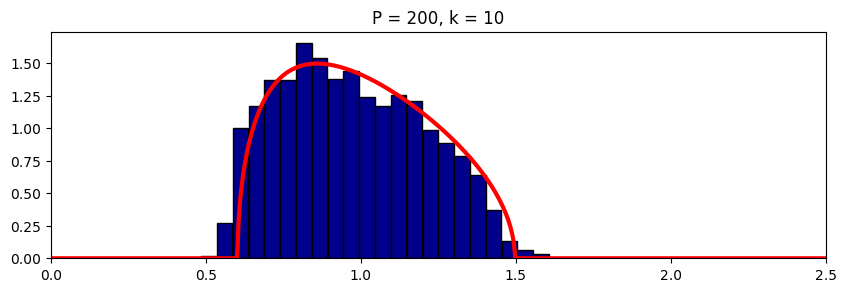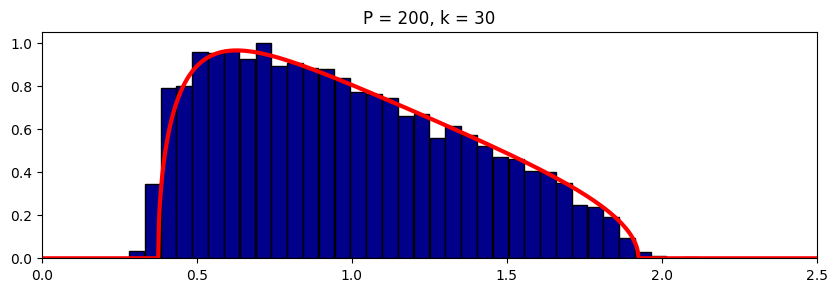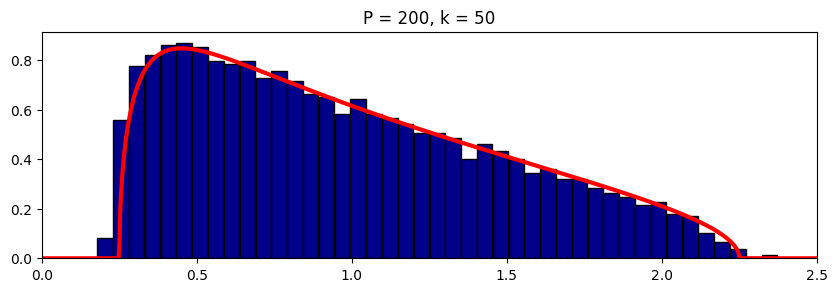WARNING: hist(...) and hist!(...) are deprecated. Use fit(Histogram,...) in StatsBase.jl instead.
in depwarn(::String, ::Symbol) at ./deprecated.jl:64
in #hist!#994(::Bool, ::Function, ::Array{Int64,1}, ::Array{Any,1}, ::LinSpace{Float64}) at ./deprecated.jl:629
in hist(::Array{Any,1}, ::LinSpace{Float64}) at ./deprecated.jl:644
in macro expansion; at /Users/gpeyre/Dropbox/github/numerical-tours/julia/NtSolutions/sparsity_6_l1_recovery/exo4.jl:22 [inlined]
in anonymous at ./<missing>:?
in include_from_node1(::String) at /Applications/Julia-0.5.app/Contents/Resources/julia/lib/julia/sys.dylib:?
in include_string(::String, ::String) at /Applications/Julia-0.5.app/Contents/Resources/julia/lib/julia/sys.dylib:?
in include_string(::Module, ::String, ::String) at /Users/gpeyre/.julia/v0.5/Compat/src/Compat.jl:577
in execute_request(::ZMQ.Socket, ::IJulia.Msg) at /Users/gpeyre/.julia/v0.5/IJulia/src/execute_request.jl:154
in invokelatest(::Function, ::ZMQ.Socket, ::Vararg{Any,N}) at /Users/gpeyre/.julia/v0.5/Compat/src/Compat.jl:587
in eventloop(::ZMQ.Socket) at /Users/gpeyre/.julia/v0.5/IJulia/src/eventloop.jl:8

In :
## Insert your code here.


Exercise 5

Estimate numerically lower bound on $\de_k^1,\de_k^2$ by Monte-Carlo sampling of sub-matrices.

In :
include("NtSolutions/sparsity_6_l1_recovery/exo5.jl")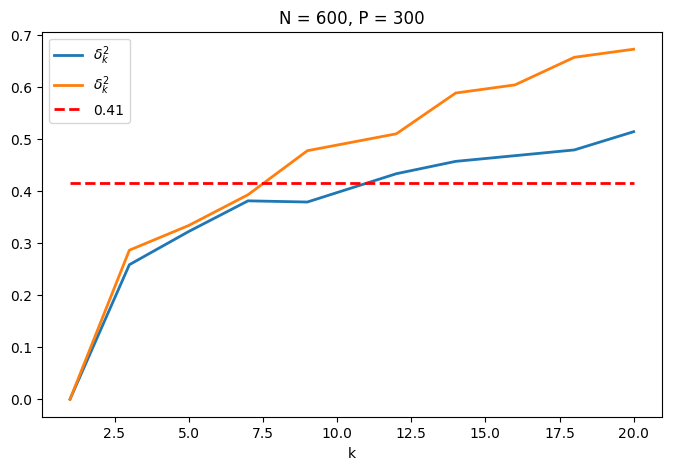In :
## Insert your code here.


## Sparse Spikes Deconvolution¶

We now consider a convolution dictionary $\Phi$. Such a dictionary is used with sparse regulariz

Second derivative of Gaussian kernel $g$ with a given variance $\si^2$.

In :
sigma = 6
g = x -> (1 - x.^2./sigma^2).*exp(-x.^2./(2*sigma^2));


Create a matrix $\Phi$ so that $\Phi x = g \star x$ with periodic boundary conditions.

In :
include("NtToolBox/src/ndgrid.jl")
P = 1024
(Y, X) = meshgrid(0:P-1, 0:P-1)
Phi = normalize(g((X - Y + P/2.)%P-P/2.));


To improve the conditionning of the dictionary, we sub-sample its atoms, so that $P = \eta N > N$.

In :
eta = 2
N = P/eta
Phi = Phi[:, 1:eta:end];


Plot the correlation function associated to the filter. Can you determine the value of the coherence $\mu(\Phi)$?

In :
c = Phi'*Phi
c = abs(c[:, Int(size(c)/2)])

figure(figsize = (8, 5))
plot(c[Base.div(length(c), 2) - 50 : Base.div(length(c), 2) + 50], ".-")
show()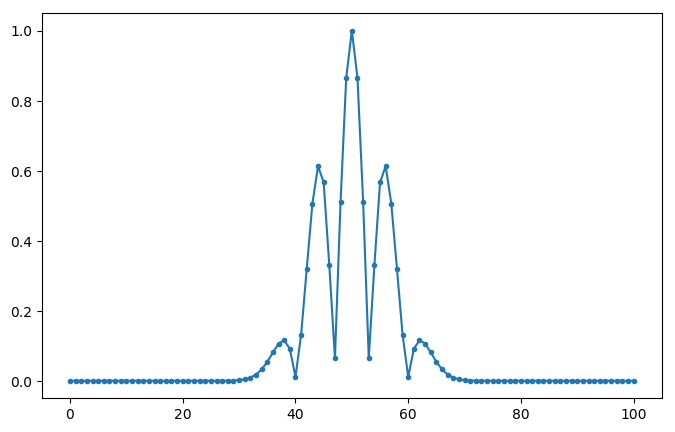Create a data a sparse $x_0$ with two diracs of opposite signes with spacing $d$.

In :
twosparse = d -> circshift([1; zeros(d, 1); -1; zeros(Int(N) - d - 2, 1)], round(N/2 - d/2));


Display $x_0$ and $\Phi x_0$.

In :
x0 = twosparse(50)

figure(figsize = (10, 7))
subplot(2, 1, 1)
plot(x0[:], "r", linewidth = 2)
subplot(2, 1, 2)
plot((Phi*x0)[:], "b", linewidth = 2)
show()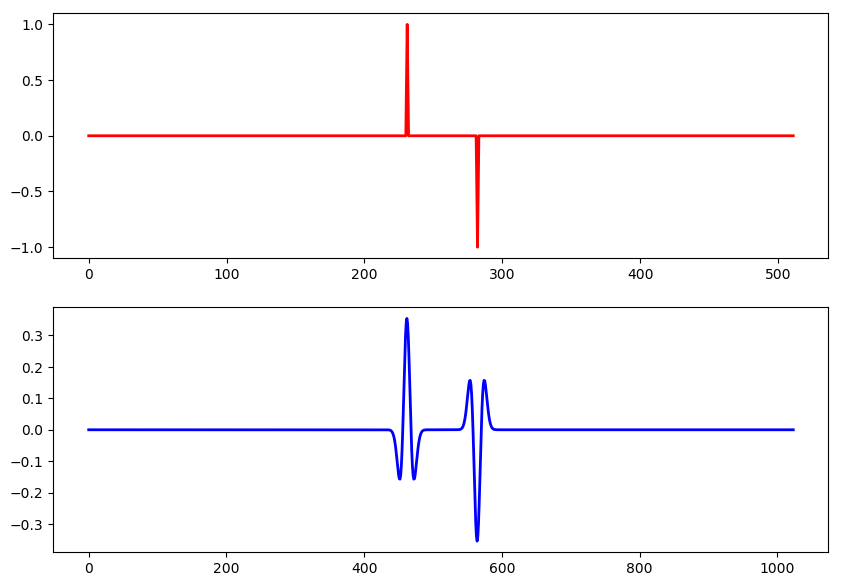Exercise 6

Plot the evolution of the criteria F, ERC and Coh as a function of $d$. Do the same plot for other signs patterns for $x_0$. Do the same plot for a Dirac comb with a varying spacing $d$.

In :
include("NtSolutions/sparsity_6_l1_recovery/exo6.jl")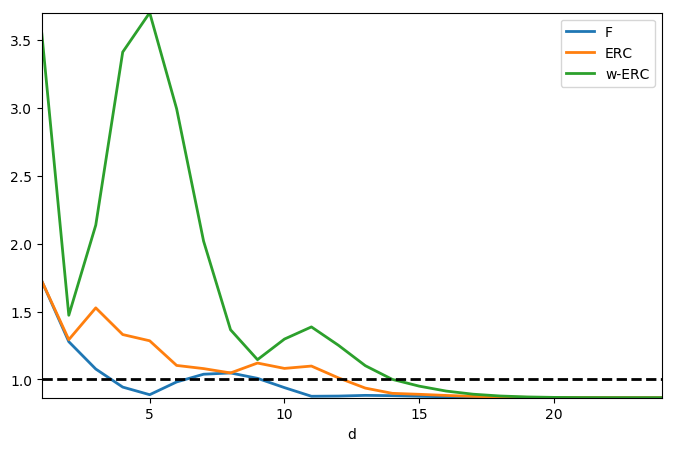In :
## Insert your code here.